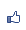[Excel VBA]如何把個股每5分鐘的成交價格記錄下來？
2009/04/30 18:25:08瀏覽23713｜回應5｜推薦0

D 欄：記錄這個時段的開始價，就是第一筆成交價。
E 欄：記錄這個時段的最高價。
F 欄：記錄這個時段的最低價。
G 欄：記錄這個時段的結束價，就是最後一筆成交價。

Excel 用小於1的正小數來表示一天從午夜開始算起的秒數，一天有24x60x60=86400秒，所以每天的第一秒, "12:00:01 AM" 用時間值表示就是 1/86400 (86400分之一, 大約 0.00115741), 第二秒就是 2/86400......依此類推。

Private Sub Worksheet_Calculate()
Dim NowDateTime, nowTime, startTime, stopTime
Dim tr As String
NowDateTime = Now '現在的時間值, 去掉整數部份,
nowTime = (NowDateTime - Int(NowDateTime)) '得到現在的時間值
startTime = Range("A6") '開盤時間, 例如: "09:00:00 AM"
stopTime = Range("A8") '收盤時間, 例如: "01:30:00 PM"
If nowTime <= startTime Then '尚未開盤
Exit Sub
ElseIf nowTime > stopTime Then '已經收盤
Exit Sub
ElseIf [A2] <> "-" And [A2] <> "###" Then '清盤的狀態, 不取其資料
tr = Int((nowTime - startTime) * 288) + 2 '每差 300 秒就換一列
If Range("D" & tr) = "" Then
Range("D" & tr) = Range("A2") '開始價
End If
If Range("E" & tr) = "" Or Range("A2") > Range("E" & tr) Then
Range("E" & tr) = Range("A2") '最高價
End If
If Range("F" & tr) = "" Or Range("A2") < Range("F" & tr) Then
Range("F" & tr) = Range("A2") '最低價
End If
Range("G" & tr) = Range("A2") '結束價
End If
End Sub

( 興趣嗜好電腦3C )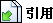引用網址：https://classic-blog.udn.com/article/trackback.jsp?uid=WayCheng&aid=2902612回應文章 選擇排序方式 　最新發表 　最舊發表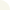ㄏ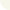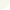2021/02/06 11:00 求檔案 感謝(howstyle666@gmail.com)george 等級：2 留言｜加入好友2020/10/06 19:39 "A2"試紙甚麼?Wilson2013/11/18 12:54

Hello :

Wilson

(a41100@gmail.com)
 ThisIsTheWay(WayCheng) 於 2017-05-19 16:15 回覆： 不，只要成交價有變動，Calculate事件就會被觸發，Calculate()就會被執行，但是我的程式這次計算出來的 tr (列號) 每隔五分鐘才會增家1，因此，五分鐘內若有多次成交價變動，後來的數值會蓋掉前面的數值。有另一種作法是 Calculate()每次執行都換列記錄，可以設一個 static 變數 ，記住現在存到第幾列，Calculate() 每執行一次就加1，這樣就會把成交價所有的變化值都記錄下來了。wearnks[Excel VBA]如何把個股每5分鐘的成交價格記錄下來？ 2010/08/31 17:10 請問：如何向您請教用法？謝謝。hk開放程式碼 2009/09/21 11:55 無法下載程式碼還是謝謝但本人已下載完成只是花點時間修改才能用